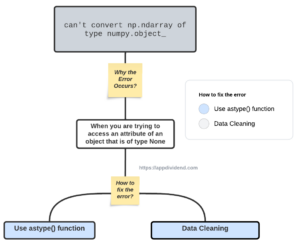# How to Fix TypeError: can’t convert np.ndarray of type numpy.object_Diagram

TypeError: can’t convert np.ndarray of type numpy.object_ error occurs when trying to “perform an operation that requires a specific data type, but your ndarray is of type numpy.object_.”

The numpy.object_ data type is a generic data type that can hold any Python object and is incompatible with operations requiring numeric types.

## Reproduce the error

``````import numpy as np

# Create a numpy array with mixed data types
arr = np.array([1, 2, '3'])

result = np.mean(arr)

print(result)``````

Output

``````TypeError: can't convert np.ndarray of type numpy.object_

# OR

numpy.core._exceptions._UFuncNoLoopError:
ufunc 'add' did not contain a loop with signature matching types
(dtype('<U21'), dtype('<U21')) -> None``````

## How to fix it?

Here are two ways to fix the TypeError: can’t convert np.ndarray of type numpy.object_.

1. Convert the data type of the ndarray using the astype() function.
2. Data Cleaning

### Solution 1: Convert the data type of the ndarray using the astype() function

``````import numpy as np

# Create a numpy array with mixed data types
arr = np.array([1, 2, '3'])

a_numeric = arr.astype(np.int64)

print(np.mean(a_numeric))
``````

Output

``2.0``

### Solution 2: Data Cleaning

``````import numpy as np

arr = np.array([1, 2, '3'])

def to_numeric(value):
try:
return int(value)
except ValueError:
try:
return float(value)
except ValueError:
return None

a_numeric_converted = np.array([to_numeric(x)
for x in arr if to_numeric(x) is not None])

# Calculate the mean of the converted array
mean_value_converted = np.mean(a_numeric_converted)
print(mean_value_converted)
``````

Output

``````2.0
``````

The inspection reveals that all the elements in the arr have the data type numpy.str_, which means they are all strings. This is why the filtering step results in an empty array, as none of the elements are recognized as int or float.

Given this, a more appropriate approach would be to attempt to convert each element to a number (either integer or float) and, if successful, include it in the numeric array.

That’s it!

## Related posts

TypeError: type numpy.ndarray doesn’t define __round__ method

TypeError: ‘numpy.ndarray’ object is not callable

TypeError: unhashable type: ‘numpy.ndarray’

TypeError: ‘numpy.float64’ object cannot be interpreted as an integer

TypeError: ‘numpy.float64’ object is not callable

TypeError: ‘float’ object is not subscriptable

This site uses Akismet to reduce spam. Learn how your comment data is processed.